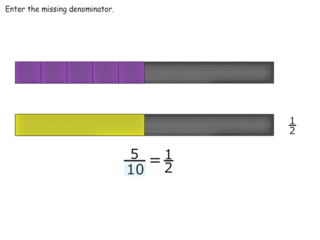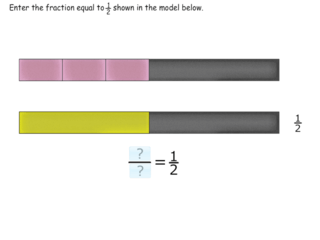# Interactive Problem Bank

•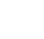Fraction Circles
•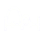Number Lines
•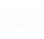Fraction Bars
•Sets
•Area Models
•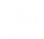Base Ten Blocks

## Equivalent Fractions

• Students use bars, number line, and real-world contexts to determine if two fractions are equivalent and to build equivalent fractions.
• Students determine how many objects in a set are equal to 1⁄2, 1⁄3, or 1⁄4 of the set.

### Model an Equivalent Fraction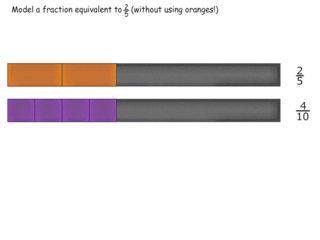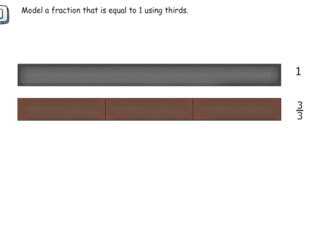### Cutting Pieces to Build Equivalents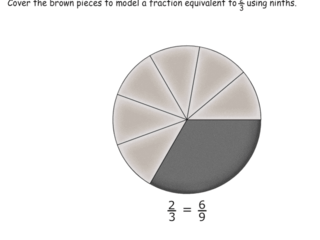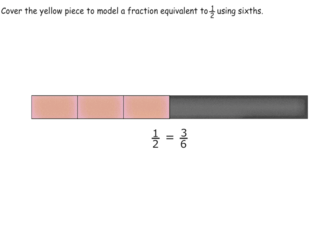### Gluing Pieces to Build Equivalents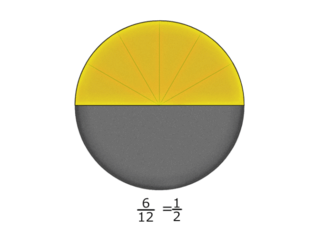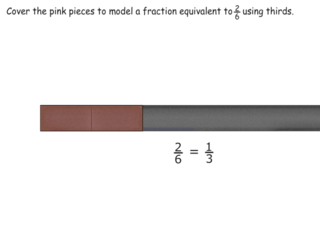### Finding Equivalent Fractions Using Models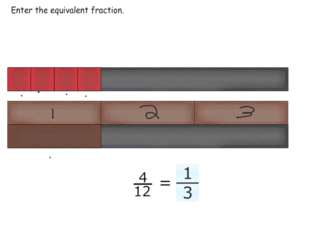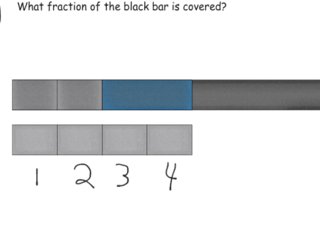### Modeling the Algorithm for Relating Equivalents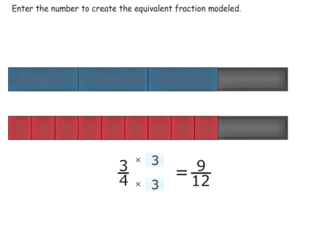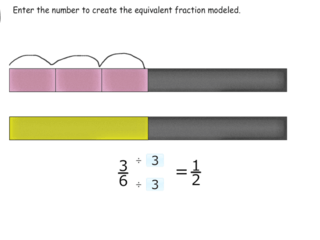### Equivalence on the Number Line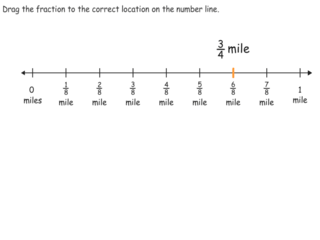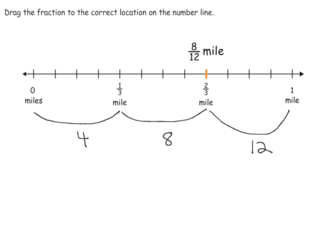### Building Equivalents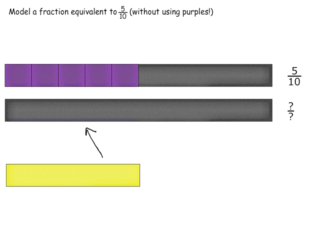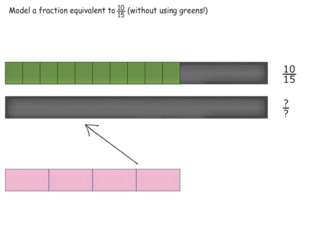### One Half of Shapes and Sets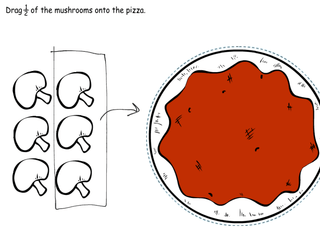### One Fourth of Shapes and Sets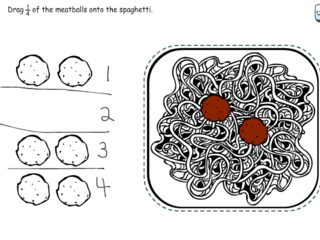### One Third of Shapes and Sets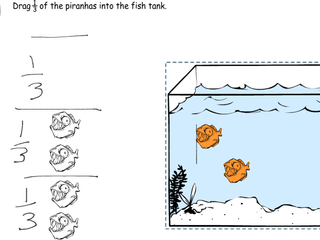### Equivalent Fractions in Partitioning Sets.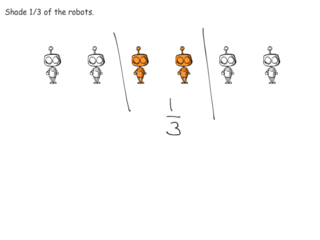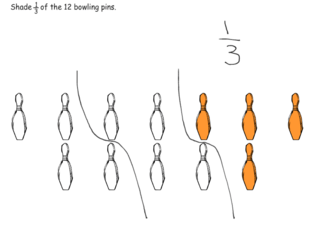### Modeling Equivalence in Context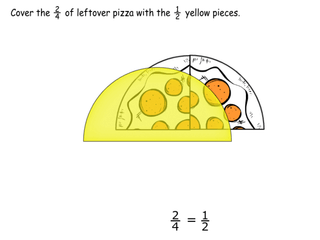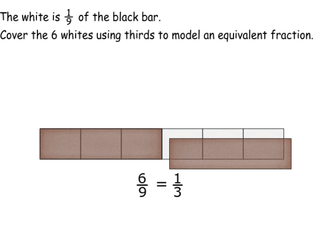### The Meaning of Equivalence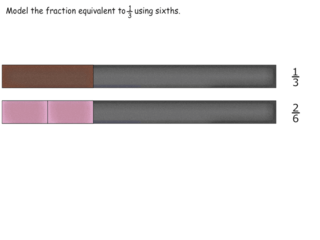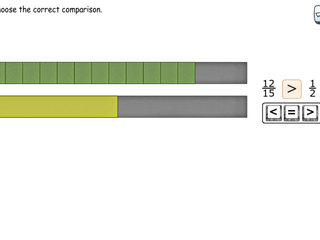### Introduction to Equivalent Fractions